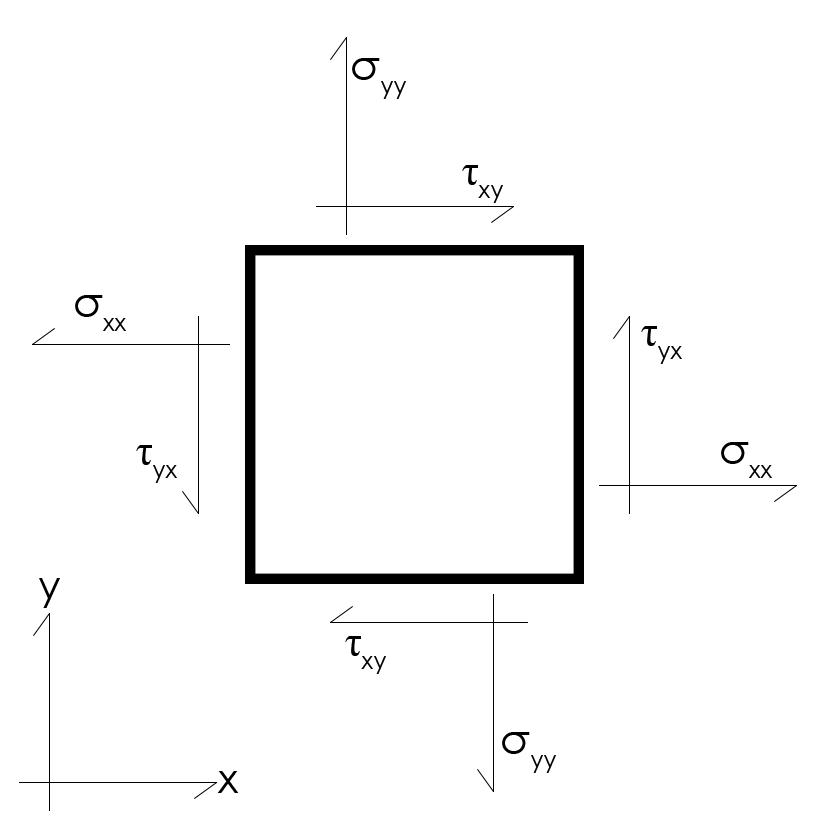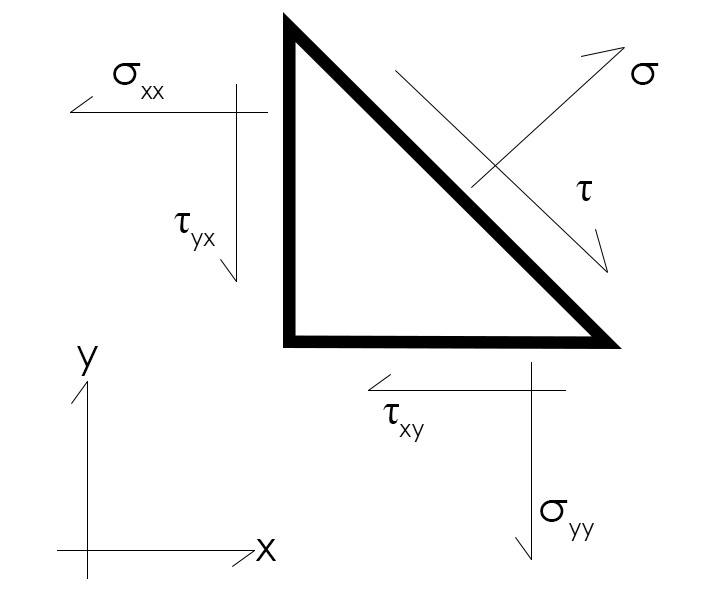# Mohr's Circle Calculator

By Rahul Dhari
Last updated: Sep 23, 2021

Mohr’s circle calculator lets you calculate the principal stresses from a 2D stress state. Utilizing the values of normal stresses and shear stresses on a body, the calculator will return to you the principal stress of the system. The stresses are regarded as one of the most fundamental aspects in designing any body or system. To this end, the calculator incorporates Mohr’s circle equations.

Read on to understand what is principal stress and how to draw Mohr's circle. The article below contains an example of Mohr's circle. Using this calculator you can calculate — principal stresses — minimum and maximum, maximum shear stresses, angle of orientation, along with von Mises and mean stress.

## What is a stress state and principal stress?

A stress state of a body is the combination of stresses at a point considering all three directions, i.e., `X`, `Y`, and `Z` or `1`, `2`, and `3`. There are three normal stresses (acting perpendicular to the face) namely, `σ11`, `σ22`, and `σ33` and six shear stresses (acting along the plane) `𝛕12`, `𝛕23`, `𝛕13`, `𝛕21`, `𝛕32`, and `𝛕31`. The stresses acting on the body are shown in the figure below.

Considering equilibrium acting on the body, the shear stresses can be reduced to three values, i.e., `𝛕12 = 𝛕21`, `𝛕13 = 𝛕31`, and `𝛕32 = 𝛕23`. Therefore, a stress state can be defined by six stresses, i.e., three normal stresses and three shear stresses. Now, if one considers only in-plane directions, the resultant stress state can be obtained by reducing the stresses, `𝛕13 = 𝛕31 = 0`, and `𝛕32 = 𝛕23 = 0`. The 2D stress state can now be defined using 3 stresses, i.e., two normal stresses (`σ11` and `σ22`) and a shear stress (`𝛕12 = 𝛕21`). This can alternatively be shown as given in the figure below (with 1 and 2 directions as x and y).## What is principal stress and How to calculate principal stress?

Consider a state at which only normal stress act on the plane. The stresses at that state are known as principal stresses. This is obtained by transforming the current stress state, i.e., reducing the shear stresses to zero.Mathematically, the principal stresses can be written using the principal stress equation:
`σ1 = ((σxx + σyy) / 2) + √(((σxx - σyy) / 2)2 + τxy2)`
`σ2 = ((σxx + σyy) / 2) - √(((σxx - σyy) / 2)2 + τxy2)`

where `σ1` and `σ2` are minimum and maximum principal stresses. Similarly, the maximum shear stress (`τmax`) for the state can be given by the equation:

`τmax = √(((σxx - σyy) / 2)2 + τxy2)`

Alternatively, maximum shear stress can also be defined using the principal stresses as:

`τmax = (σ1 - σ2) / 2`

and the mean stress (`σmean`) is written as:

`σmean = (σxx + σyy) / 2`

The angle of orientation, θ is given by:

`2θ = tan-1(2*τxy/(σxx - σyy))`

The above set of equations help you in drawing Mohr's circle and vice versa. The above equations can also be derived or obtained using geometrical approach as given in the following section.

## Utilizing the Mohr's circle to estimate principal stress

In order to utilize Mohr's circle to estimate principle stress, first, you need to understand what is Mohr's circle and how to draw a Mohr's circle. A Mohr's circle is a graphical representation of a stress state and is used to perform stress transformations. To draw a Mohr's circle for a given 2D stress state with normal stresses (`σxx` and `σyy`) and shear stresses (`τxy` and `τyx`):

1. Plot the coordinates (`σyy`,`τxy`) and (`σxx`,`-τxy`) as points `A` and `B`, respectively with `σ` as X axis and `τ` as Y axis.
2. Join the points, `A` and `B` to obtain diameter `AB`.
3. Find the center of the circle, `O`, i.e., the point at which line `AB` intersects the X-axis.
4. Draw the circle with the center point as `O`.
5. The points at which the circle intersects `X`-axis are the principal stresses.

## How to use Mohr's circle calculator.

Follow the steps below to use the principal stress formula and Mohr's circle calculator.

1. Enter the normal stress in X direction, `σxx`.
2. Insert the normal stress in Y direction, `σyy`.
3. Fill in the shear stress, `τxy`.
4. Mohr's circle calculator will now use the principal stress equations to calculate maximum and minimum principal stresses, maximum shear stress, angle of orientation, von Mises and mean stress.

## FAQ

### What is a stress state?

A stress state of a body is the combination of stresses at a point considering all three directions, i.e., `X`, `Y`, and `Z` or `1`, `2`, and `3`.

### What is Mohr's circle?

Mohr's circle is the 2D graphical representation of the stress state and can be used for the purpose of stress transformation.

### What is principal stress?

Principal stresses are defined as the normal stress acting on a plane when there's no shear stress involved.

### How to calculate principal stress?

Principal stresses can be calculated using the principal stress formula:

`σ1 = ((σxx + σyy) / 2) + √(((σxx - σyy) / 2)2 + τxy2)`
`σ2 = ((σxx + σyy) / 2) - √(((σxx - σyy) / 2)2 + τxy2)`

where `σ1` and `σ2` are minimum and maximum principal stresses.

Rahul Dhari
Normal stresses
Normal stress in X direction (σ_xx)
psi
Normal stress in Y direction (σ_yy)
psi
Shear stresses
Shear stress (𝛕_xy)
psi
Shear stress (𝛕_yx)
psi
Principal stresses
Maximum principal stress (σ_1)
psi
Minimum principal stress (σ_2)
psi
Other results
Maximum shear stress (𝛕_max)
psi
von Mises stress (σ_mises)
psi
Angle of orientation (θ)
Mean stress (σ_m)
psi
People also viewed…

### Car crash force

With this car crash calculator, you can find out how dangerous are car crashes.

### Centrifugal force

Use our centrifugal force calculator to determine the force acting on a rotating object.

### Photon energy

The Photon energy calculator computes the energy of a single photon from its wavelength or frequency.